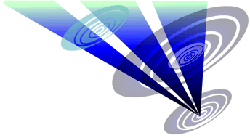Reconstruction of the 2016 Silbury Hill (Phase 2) formation 1.Draw a circle. Draw and extend the horizontal and vertical centerlines. 2.Construct the inscribed pentagon (regular 5-sided polygon) of circle 1, pointing up. 3.Construct the inscribed pentagon of circle 1, pointing down. 4.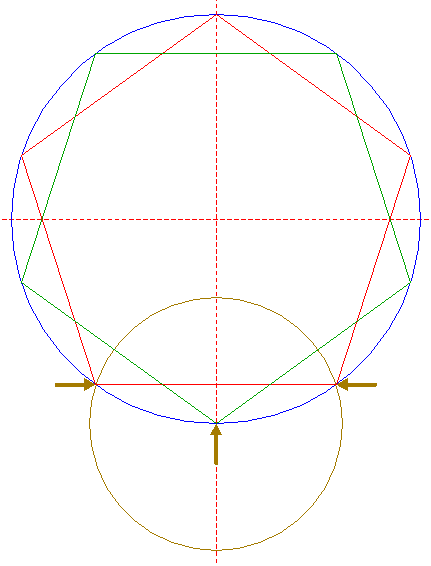Construct a circle centered at the lower angular point of pentagon 3, passing through the two lower angular points of pentagon 2. 5.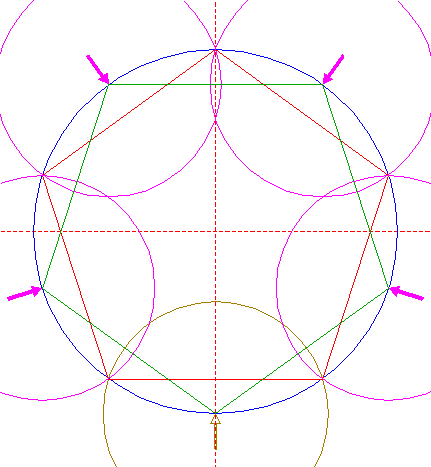Copy circle 4 four times, to the other angular points of pentagon 3. 6.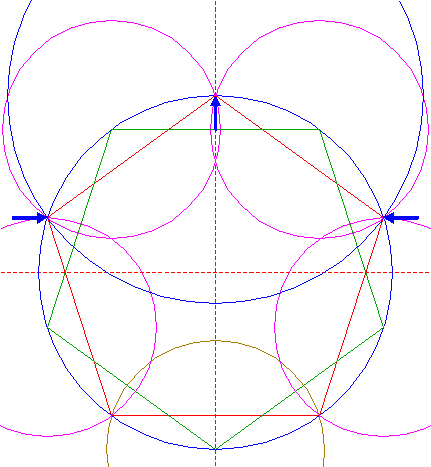Construct a circle centered at the upper angular point of pentagon 2, passing through its upper lefthand and upper righthand angular points. 7.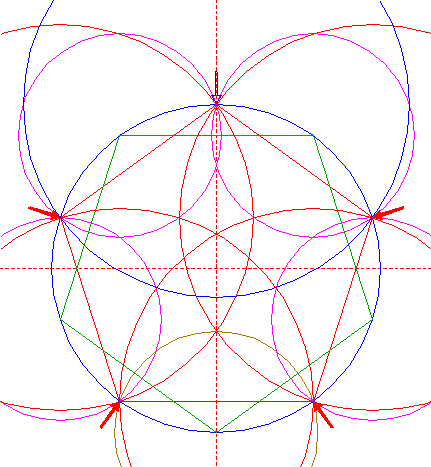Copy circle 6 four times, to the other angular points of pentagon 2. 8.Construct a circle concentric to circle 1, tangent to circle 6 at the lower side. 9.Construct a circle centered at the intersection of the upper side of pentagon 3 and the vertical centerline, passing through the upper lefthand and upper righthand angular points of pentagon 2. 10.Construct a circle concentric to circle 1, tangent to circle 9 at the lower side. 11.Construct the inscribed pentagon of a circle, concentric to circle 1 and tangent to circle 4 at the upper side, pointing down. 12.Construct the inscribed circle of pentagon 11. 13.Copy circle 9 to the center of circle 1. 14.Construct a circle centered at the upper intersection of circle 12 and the vertical centerline, tangent to circle 10 at the upper side. 15.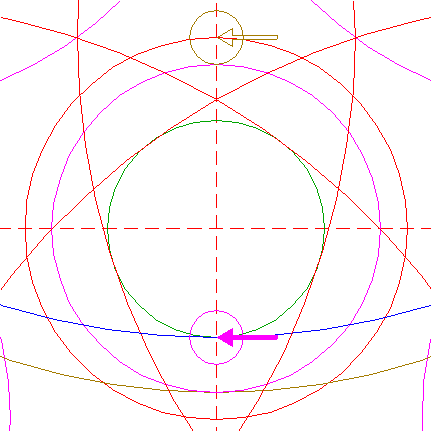Copy circle 14 to the lower intersection of circle 6 and the vertical centerline. 16.Construct a circle concentric to circle 6, tangent to circle 15 at the lower side. 17.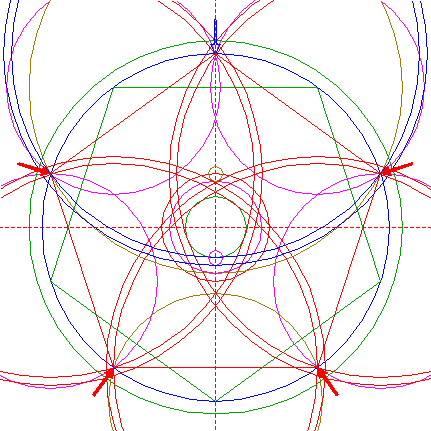Copy circle 16 four times, to the centers of circles 7. 18.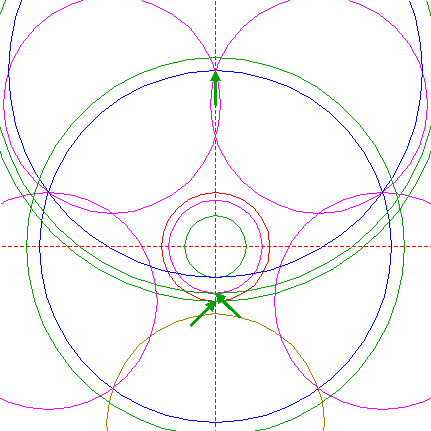For clarity reasons, some of the results of previous steps are temporarily removed.Construct two circles concentric to circle 6, tangent to circles 10 and 12 respectively, both at the lower sides. 19.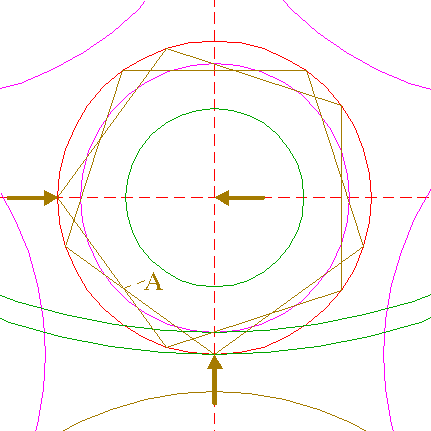Construct two inscribed pentagons of circle 12, one pointing down, one to the left. Call the innermost lower lefthand intersection of these pentagons point A. 20.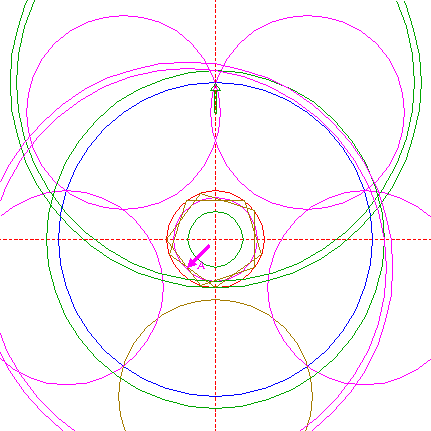Copy circles 18 to point A. See detail.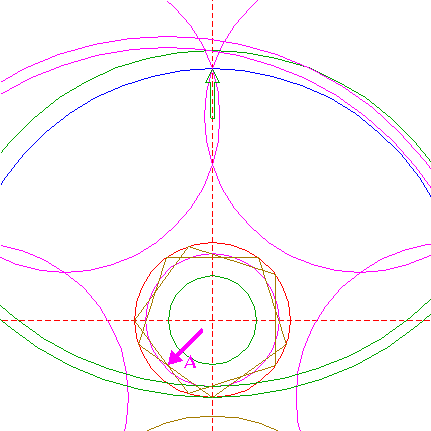21.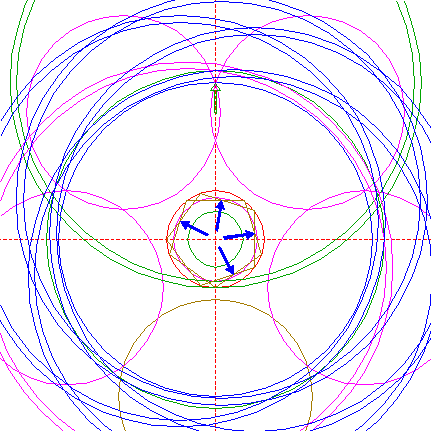Copy circles 18 four more times, to the other corresponding intersections of pentagons 19, as shown. See detail.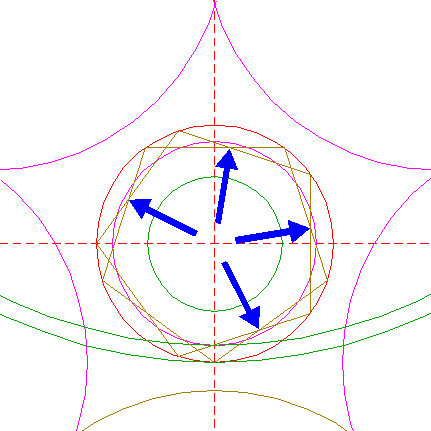22.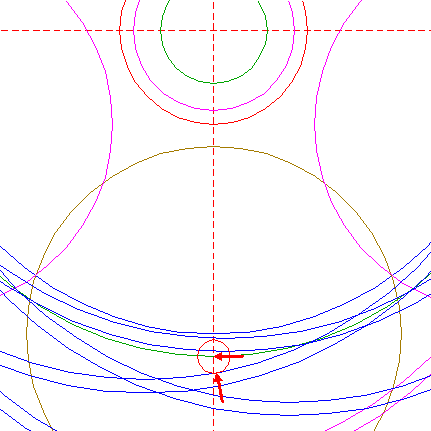Construct a circle centered at the lower intersection of circle 13 and the vertical centerline, tangent to the nearest circle 21 below this point, as shown. 23.Construct a circle concentric to circle 22, tangent to the next nearest circle 21 below, as shown. 24.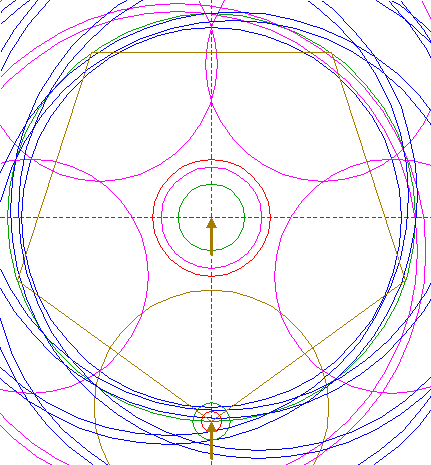Construct the inscribed pentagon of circle 13, pointing down. 25.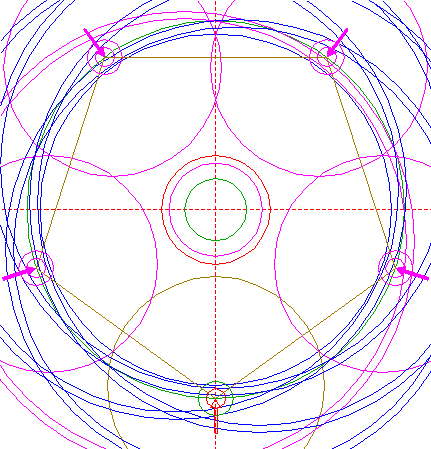Copy circles 22 and 23 four times, to the other angular points of pentagon 24. 26.Construct a circle centered at the lower intersection of the outer circle 20 and the vertical centerline, tangent to the nearest circle 21, as shown. See detail.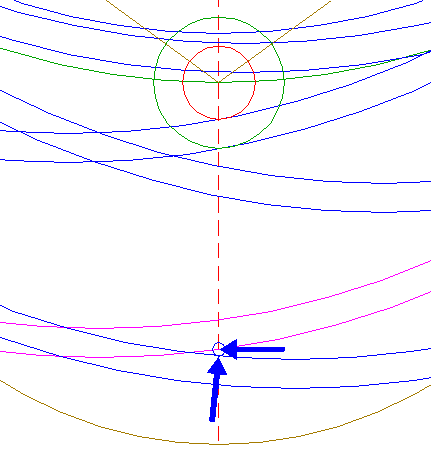27.Construct a circle concentric to circle 26, tangent to the next nearest circle 21, as shown. See detail.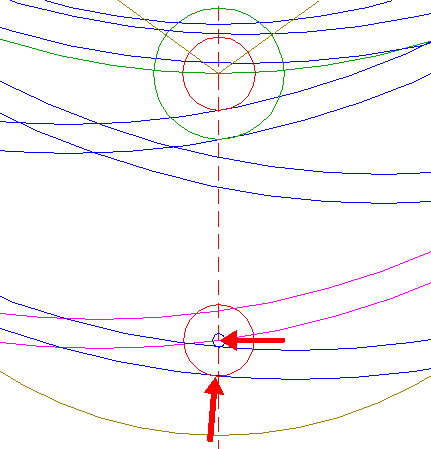28.Construct a pentagon concentric to circle 1, with one angular point at the center of circle 26. 29.Copy circles 26 and 27 four times, to the other angular points of pentagon 28. 30.Circles 4, 5, 6, 7, 8, 10, 12, 13, 16, 17, 20, 21, 22, 23, 25, 26, 27, and 29, are used for the final reconstruction. 31.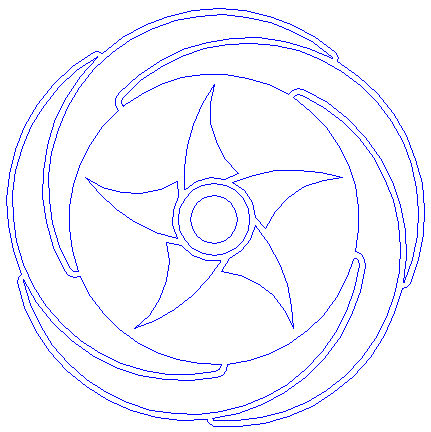Remove all parts not visible within the formation itself. 32.Colour all areas corresponding to standing... 33....or to flattened crop, and finish the reconstruction of the 2016 Silbury Hill (Phase 2) formation. 34.The final result, matched with the aerial image.